Next: Basic Equations of Ideal Up: Magnetohydrodynamics Previous: Magnetohydrodynamics   Contents

## Flux Freezing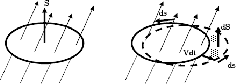An important nature of the ideal MHD is derived from equatio (B.4), that is, the matter and the magnetic field is coupled with each other. Consider a gas parcel threaded with the magnetic field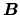. The magnetic flux anchored to the gas parcel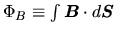is changes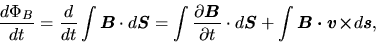(B.5)

where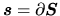. The first term of the lhs of the equation comes from a time derivative of the magnetic flux density, while the last term represents the change of the integral region due to gas motion (see Fig.B.1). (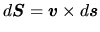) Using the Stokes' theorem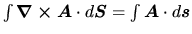, the last term is reduced to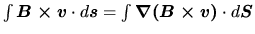. Finally, we obtain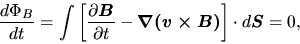(B.6)

where we used equation (B.4). This means that the magnetic flux anchored to a gas parcel does not change in the ideal MHD regime.Next: Basic Equations of Ideal Up: Magnetohydrodynamics Previous: Magnetohydrodynamics   Contents
Kohji Tomisaka 2007-07-08